## Preface

After you have acquired the data, you should do the following:

• Diagnose data quality.
• If there is a problem with data quality,
• The data must be corrected or re-acquired.
• Explore data to understand the data and find scenarios for performing the analysis.
• Derive new variables or perform variable transformations.

The dlookr package makes these steps fast and easy:

• Performs an data diagnosis or automatically generates a data diagnosis report.
• Discover data in a variety of ways, and automatically generate EDA(exploratory data analysis) report.
• Imputate missing values and outliers, resolve skewed data, and binarize continuous variables into categorical variables. And generates an automated report to support it.

This document introduces Data Quality Diagnosis methods provided by the dlookr package. You will learn how to diagnose the quality of `tbl_df` data that inherits from data.frame and `data.frame` with functions provided by dlookr.

dlookr synergy with `dplyr` increases. Particularly in data exploration and data wrangle, it increases the efficiency of the `tidyverse` package group.

## Supported data structures

Data diagnosis supports the following data structures.

• data frame : data.frame class.
• data table : tbl_df class.
• table of DBMS : table of the DBMS through tbl_dbi.
• Using dplyr backend for any DBI-compatible database.

## Data: nycflights13

To illustrate basic use of the dlookr package, use the `flights` data from the `nycflights13` package. The `flights` data frame is data about departure and arrival on all flights departing from NYC in 2013.

``````library(nycflights13)
dim(flights)
 336776     19
flights
# A tibble: 336,776 x 19
year month   day dep_time sched_dep_time dep_delay arr_time
<int> <int> <int>    <int>          <int>     <dbl>    <int>
1  2013     1     1      517            515         2      830
2  2013     1     1      533            529         4      850
3  2013     1     1      542            540         2      923
4  2013     1     1      544            545        -1     1004
# … with 336,772 more rows, and 12 more variables: sched_arr_time <int>,
#   arr_delay <dbl>, carrier <chr>, flight <int>, tailnum <chr>,
#   origin <chr>, dest <chr>, air_time <dbl>, distance <dbl>, hour <dbl>,
#   minute <dbl>, time_hour <dttm>
``````

## Data diagnosis

dlookr aims to diagnose the data and to select variables that can not be used for data analysis or to find the variables that need to be calibrated.:

• `diagnose()` provides basic diagnostic information for variables.
• `diagnose_category()` provides detailed diagnostic information for categorical variables.
• `diagnose_numeric()` provides detailed diagnostic information for numeric variables.
• `diagnose_outlier()` and `plot_outlier()` provide information and visualization of outliers.

### General diagnosis of all variables with `diagnose()`

`diagnose()` allows you to diagnosis a variables in a data frame. Like function of dplyr, the first argument is the tibble (or data frame). The second and subsequent arguments refer to variables within that data frame.

The variables of the `tbl_df` object returned by `diagnose ()` are as follows.

• `variables` : variable name
• `types` : the data type of the variable
• `missing_count` : number of missing values
• `missing_percent` : percentage of missing values
• `unique_count` : number of unique values
• `unique_rate` : rate of unique value. unique_count / number of observation

For example, we can diagnose all variables in `flights`:

``````diagnose(flights)
# A tibble: 19 x 6
variables types   missing_count missing_percent unique_count unique_rate
<chr>     <chr>           <int>           <dbl>        <int>       <dbl>
1 year      integer             0            0               1  0.00000297
2 month     integer             0            0              12  0.0000356
3 day       integer             0            0              31  0.0000920
4 dep_time  integer          8255            2.45         1319  0.00392
# … with 15 more rows
``````
• `Missing Value(NA)` : Variables with very large missing values, ie those with a `missing_percent` close to 100, should be excluded from the analysis.
• `Unique value` : Variables with a unique value (`unique_count` = 1) are considered to be excluded from data analysis. And if the data type is not numeric (integer, numeric) and the number of unique values is equal to the number of observations (unique_rate = 1), then the variable is likely to be an identifier. Therefore, this variable is also not suitable for the analysis model.

`year` can be considered not to be used in the analysis model since `unique_count` is 1. However, you do not have to remove it if you configure `date` as a combination of `year`, `month`, and `day`.

For example, we can diagnose only a few selected variables:

``````# Select columns by name
diagnose(flights, year, month, day)
# A tibble: 3 x 6
variables types   missing_count missing_percent unique_count unique_rate
<chr>     <chr>           <int>           <dbl>        <int>       <dbl>
1 year      integer             0               0            1  0.00000297
2 month     integer             0               0           12  0.0000356
3 day       integer             0               0           31  0.0000920
# Select all columns between year and day (inclusive)
diagnose(flights, year:day)
# A tibble: 3 x 6
variables types   missing_count missing_percent unique_count unique_rate
<chr>     <chr>           <int>           <dbl>        <int>       <dbl>
1 year      integer             0               0            1  0.00000297
2 month     integer             0               0           12  0.0000356
3 day       integer             0               0           31  0.0000920
# Select all columns except those from year to day (inclusive)
diagnose(flights, -(year:day))
# A tibble: 16 x 6
variables    types missing_count missing_percent unique_count unique_rate
<chr>        <chr>         <int>           <dbl>        <int>       <dbl>
1 dep_time     inte…          8255            2.45         1319     0.00392
2 sched_dep_t… inte…             0            0            1021     0.00303
3 dep_delay    nume…          8255            2.45          528     0.00157
4 arr_time     inte…          8713            2.59         1412     0.00419
# … with 12 more rows
``````

By using dplyr, variables including missing values can be sorted by the weight of missing values.:

``````flights %>%
diagnose() %>%
select(-unique_count, -unique_rate) %>%
filter(missing_count > 0) %>%
arrange(desc(missing_count))
# A tibble: 6 x 4
variables types   missing_count missing_percent
<chr>     <chr>           <int>           <dbl>
1 arr_delay numeric          9430            2.80
2 air_time  numeric          9430            2.80
3 arr_time  integer          8713            2.59
4 dep_time  integer          8255            2.45
# … with 2 more rows
``````

### Diagnosis of numeric variables with `diagnose_numeric()`

`diagnose_numeric()` diagnoses numeric(continuous and discrete) variables in a data frame. Usage is the same as `diagnose()` but returns more diagnostic information. However, if you specify a non-numeric variable in the second and subsequent argument list, the variable is automatically ignored.

The variables of the `tbl_df` object returned by `diagnose_numeric()` are as follows.

• `min` : minimum value
• `Q1` : ¼ quartile, 25th percentile
• `mean` : arithmetic mean
• `median` : median, 50th percentile
• `Q3` : ¾ quartile, 75th percentile
• `max` : maximum value
• `zero` : number of observations with a value of 0
• `minus` : number of observations with negative numbers
• `outlier` : number of outliers

Applying the summary () function to a data frame can help you figure out the distribution of data by printing `min`, `Q1`, `mean`, `median`, `Q3`, and `max` give. However, the result is that analysts can only look at it with eyes. However, returning such information as a data frame structure like `tbl_df` widens the scope of utilization.

`zero`, `minus`, and `outlier` are useful for diagnosing the integrity of data. For example, numerical data in some cases may not have 0 or a negative number. Since the hypothetical numeric variable 'employee salary' can not have a negative or zero value, you should check for zero or negative numbers in the data diagnosis process.

`diagnose_numeric()` can diagnose all numeric variables of `flights` as follows.:

``````diagnose_numeric(flights)
# A tibble: 14 x 10
variables   min    Q1    mean median    Q3   max  zero minus outlier
<chr>     <dbl> <dbl>   <dbl>  <dbl> <dbl> <dbl> <int> <int>   <int>
1 year       2013  2013 2013      2013  2013  2013     0     0       0
2 month         1     4    6.55      7    10    12     0     0       0
3 day           1     8   15.7      16    23    31     0     0       0
4 dep_time      1   907 1349.     1401  1744  2400     0     0       0
# … with 10 more rows
``````

If a numeric variable can not logically have a negative or zero value, it can be used with `filter()` to easily find a variable that does not logically match:

``````diagnose_numeric(flights) %>%
filter(minus > 0 | zero > 0)
# A tibble: 3 x 10
variables   min    Q1  mean median    Q3   max  zero  minus outlier
<chr>     <dbl> <dbl> <dbl>  <dbl> <dbl> <dbl> <int>  <int>   <int>
1 dep_delay   -43    -5 12.6      -2    11  1301 16514 183575   43216
2 arr_delay   -86   -17  6.90     -5    14  1272  5409 188933   27880
3 minute        0     8 26.2      29    44    59 60696      0       0
``````

### Diagnosis of categorical variables with `diagnose_category()`

`diagnose_category()` diagnoses the categorical(factor, ordered, character) variables of a data frame. The usage is similar to `diagnose ()` but returns more diagnostic information. If you specify a non-categorical variable in the second and subsequent argument list, the variable is automatically ignored. The top argument specifies the number of levels to return per variable. The default value is 10, which returns the top 10 level. Of course, if the number of levels is less than 10, all levels are returned.

The variables of the `tbl_df` object returned by `diagnose_category()` are as follows.

• `variables` : variable names
• `levels`: level names
• `N` : Number of observation
• `freq` : Number of observation at the levles
• `ratio` : Percentage of observation at the levles
• `rank` : Rank of occupancy ratio of levels

`diagnose_category()` can diagnose all categorical variables of `flights` as follows.:

``````diagnose_category(flights)
# A tibble: 33 x 6
variables levels      N  freq ratio  rank
<chr>     <chr>   <int> <int> <dbl> <int>
1 carrier   UA     336776 58665  17.4     1
2 carrier   B6     336776 54635  16.2     2
3 carrier   EV     336776 54173  16.1     3
4 carrier   DL     336776 48110  14.3     4
# … with 29 more rows
``````

In collaboration with `filter()` in the `dplyr` package, we can see that the `tailnum` variable is ranked in top 1 with 2,512 missing values in the case where the missing value is included in the top 10:

``````diagnose_category(flights) %>%
filter(is.na(levels))
# A tibble: 1 x 6
variables levels      N  freq ratio  rank
<chr>     <chr>   <int> <int> <dbl> <int>
1 tailnum   <NA>   336776  2512 0.746     1
``````

The following returns a list of levels less than or equal to 0.01%. It should be noted that the top argument has a generous specification of 500. If you use the default value of 10, values below 0.01% would not be included in the list:

``````flights %>%
diagnose_category(top = 500)  %>%
filter(ratio <= 0.01)
# A tibble: 10 x 6
variables levels      N  freq   ratio  rank
<chr>     <chr>   <int> <int>   <dbl> <int>
1 carrier   OO     336776    32 0.00950    16
2 dest      JAC    336776    25 0.00742    97
3 dest      PSP    336776    19 0.00564    98
4 dest      EYW    336776    17 0.00505    99
# … with 6 more rows
``````

In the analytical model, it is also possible to consider removing the small percentage of observations in the observations or joining them together.

### Diagnosing outliers with `diagnose_outlier()`

`diagnose_outlier()` diagnoses the outliers of the numeric (continuous and discrete) variables of the data frame. The usage is the same as `diagnose()`.

The variables of the `tbl_df` object returned by `diagnose_outlier()` are as follows.

• `outliers_cnt` : Count of outliers
• `outliers_ratio` : Percent of outliers
• `outliers_mean` : Arithmetic Average of outliers
• `with_mean` : Arithmetic Average of with outliers
• `without_mean` : Arithmetic Average of without outliers

`diagnose_outlier()` can diagnose anomalies of all numeric variables of `flights` as follows:

``````diagnose_outlier(flights)
# A tibble: 14 x 6
variables outliers_cnt outliers_ratio outliers_mean with_mean
<chr>            <int>          <dbl>         <dbl>     <dbl>
1 year                 0              0           NaN   2013
2 month                0              0           NaN      6.55
3 day                  0              0           NaN     15.7
4 dep_time             0              0           NaN   1349.
# … with 10 more rows, and 1 more variable: without_mean <dbl>
``````

Numeric variables that contain anomalies are easily found with `filter()`.:

``````diagnose_outlier(flights) %>%
filter(outliers_cnt > 0)
# A tibble: 5 x 6
variables outliers_cnt outliers_ratio outliers_mean with_mean
<chr>            <int>          <dbl>         <dbl>     <dbl>
1 dep_delay        43216      12.8               93.1     12.6
2 arr_delay        27880       8.28             121.       6.90
3 flight               1       0.000297        8500     1972.
4 air_time          5448       1.62             400.     151.
# … with 1 more row, and 1 more variable: without_mean <dbl>
``````

The following is a list of numeric variables with anomalies greater than 5%.:

``````diagnose_outlier(flights) %>%
filter(outliers_ratio > 5) %>%
mutate(rate = outliers_mean / with_mean) %>%
arrange(desc(rate)) %>%
select(-outliers_cnt)
# A tibble: 2 x 6
variables outliers_ratio outliers_mean with_mean without_mean  rate
<chr>              <dbl>         <dbl>     <dbl>        <dbl> <dbl>
1 arr_delay           8.28         121.       6.90       -3.69  17.5
2 dep_delay          12.8           93.1     12.6         0.444  7.37
``````

If the outlier is larger than the average of all observations, it may be desirable to replace or remove the outlier in the data analysis process.

### Visualization of outliers using `plot_outlier()`

`plot_outlier()` visualizes outliers of numarical variables(continious and discrete) of data.frame. Usage is the same `diagnose()`.

The plot derived from the numerical data diagnosis is as follows.

• With outliers box plot
• Without outliers box plot
• With outliers histogram
• Without outliers histogram

`plot_outlier()` can visualize an anomaly in the `arr_delay` variable of `flights` as follows:

``````flights %>%
plot_outlier(arr_delay)
``````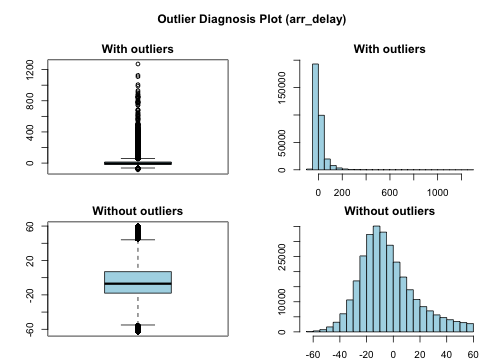Use the function of the dplyr package and `plot_outlier()` and `diagnose_outlier()` to visualize anomaly values of all numeric variables with an outlier ratio of 0.5% or more.:

``````flights %>%
plot_outlier(diagnose_outlier(flights) %>%
filter(outliers_ratio >= 0.5) %>%
select(variables) %>%
unlist())
``````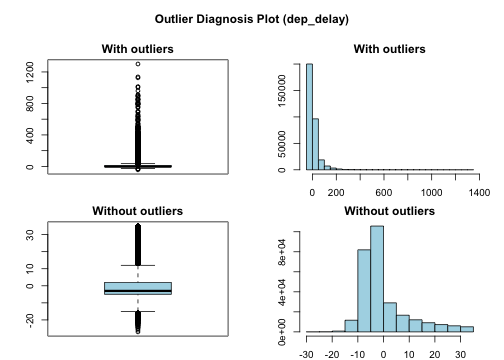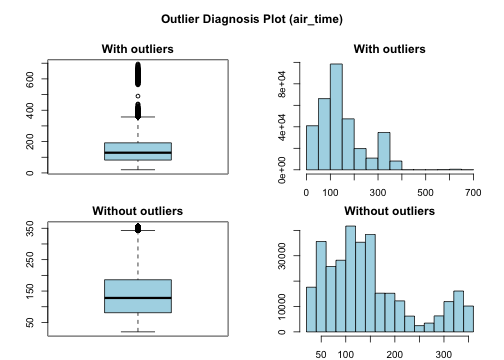You should look at the visualization results and decide whether to remove or replace the outliers. In some cases, it is important to consider removing the variables that contain anomalies from the data analysis model.

In the visualization results, `arr_delay` has similar distributions to the normal distribution of the observed values. In the case of linear models, we can also consider removing or replacing anomalies. And `air_time` shows a roughly similar distribution before and after removing anomalies.

### Create a diagnostic report using `diagnose_report()`

`diagnose_report()` performs data diagnosis of all variables of object inherited from data.frame(`tbl_df`, `tbl`, etc) or data.frame.

diagnose_report() writes the report in two formats:

• Latex based pdf file
• html file

The contents of the report are as follows.:

• Diagnose Data
• Overview of Diagnosis
• List of all variables quality
• Diagnosing Missing Data
• Diagnosis of unique data(Text and Category)
• Diagnosis of unique data(Numerical)
• Detailed data diagnosis
• Diagnosis of categorical variables
• Diagnosis of numerical variables
• List of numerical diagnosis (zero)
• List of numerical diagnosis (minus)
• Diagnose Outliers
• Overview of Diagnosis
• Diagnosis of numerical variable outliers
• Detailed outliers diagnosis

The follwing creates a quality diagnostic report for flights, a `tbl_df` class object. The file format is pdf and file name is `DataDiagnosis_Report.pdf`.

``````flights %>%
diagnose_report()
``````

The following script creates an html report named `DataDiagnosis_Report.html`.

``````flights %>%
diagnose_report(output_format = "html")
``````

The following generates an HTML report named `Diagn.html`.

``````flights %>%
diagnose_report(output_format = "html", output_file = "Diagn.html")
``````

The `Data Diagnostic Report` is an automated report intended to aid in the data diahnosis process. It judged whether the data is supplemented or reacquired by referring to the report results.

### Diagnostic report contents

#### Contents of pdf file

• The cover of the report is shown in the following figure.: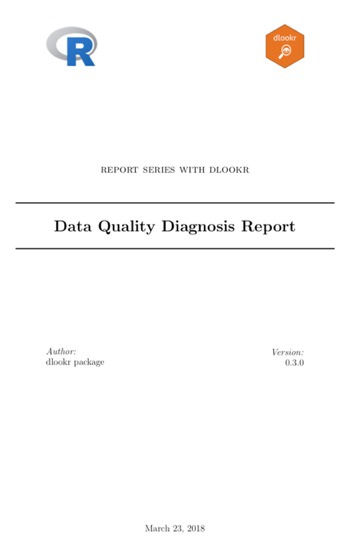• The contents of the report are shown in the following figure.: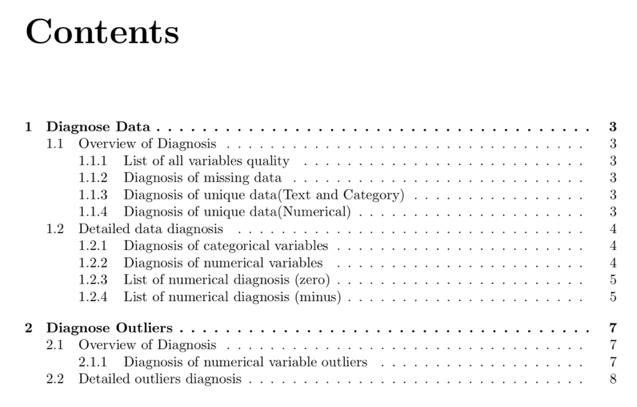• Most information is represented in the report as a table. An example of a table is shown in the following figure.: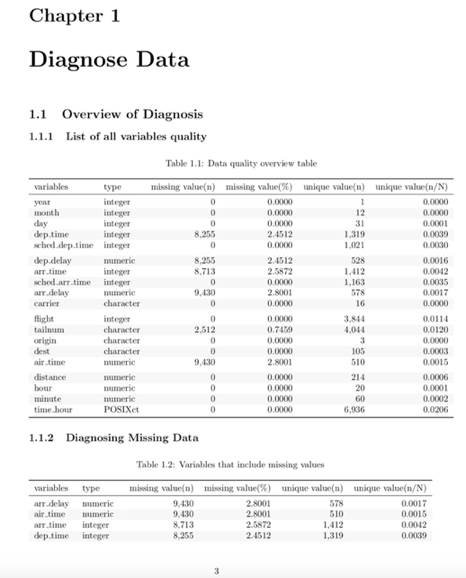• In the data diagnosis report, the outlier diagnostic contents include visualization results. The result is shown in the following figure.: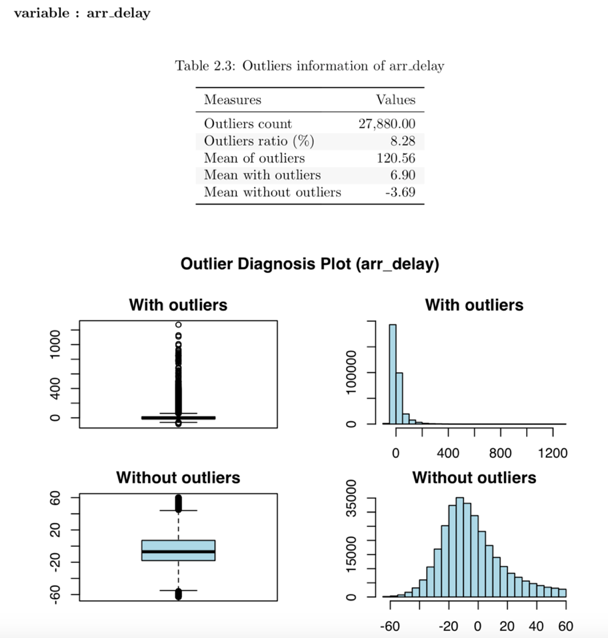#### Contents of html file

• The title and contents of the report are shown in the following figure.: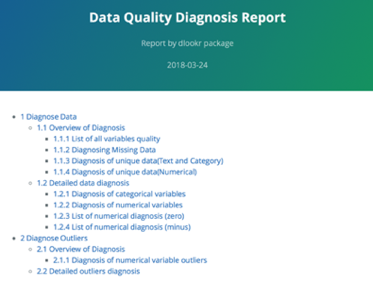• Most of the information is represented in tables in reports. An example of a table in an html file is shown in the following figure.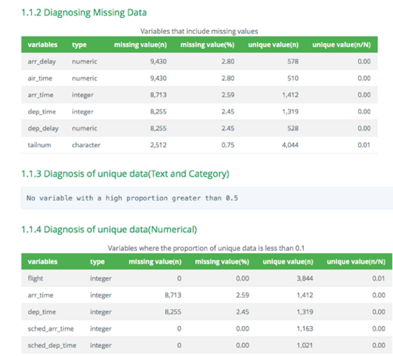• In the data diagnosis report, the outlier diagnostic contents include visualization results. The result of the html file is shown in the following figure.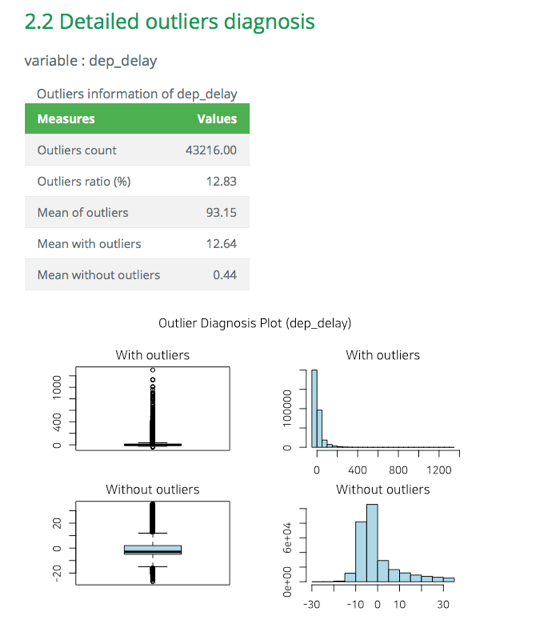## Diagnosing tables in DBMS

The DBMS table diagnostic function supports In-database mode that performs SQL operations on the DBMS side. If the size of the data is large, using In-database mode is faster.

It is difficult to obtain anomaly or to implement the sampling-based algorithm in SQL of DBMS. So some functions do not yet support In-database mode. In this case, it is performed in In-memory mode in which table data is brought to R side and calculated. In this case, if the data size is large, the execution speed may be slow. It supports the collect_size argument, which allows you to import the specified number of samples of data into R.

• In-database support fuctions
• `diagonse()`
• `diagnose_category()`
• In-database not support fuctions
• `diagnose_numeric()`
• `diagnose_outlier()`
• `plot_outlier()`
• `diagnose_report()`

### Preparing table data

Copy the `carseats` data frame to the SQLite DBMS and create it as a table named `TB_CARSEATS`. Mysql/MariaDB, PostgreSQL, Oracle DBMS, etc. are also available for your environment.

``````if (!require(DBI)) install.packages('DBI')
if (!require(RSQLite)) install.packages('RSQLite')
if (!require(dplyr)) install.packages('dplyr')
if (!require(dbplyr)) install.packages('dbplyr')

library(dplyr)

carseats <- ISLR::Carseats
carseats[sample(seq(NROW(carseats)), 20), "Income"] <- NA
carseats[sample(seq(NROW(carseats)), 5), "Urban"] <- NA

# connect DBMS
con_sqlite <- DBI::dbConnect(RSQLite::SQLite(), ":memory:")

# copy carseats to the DBMS with a table named TB_CARSEATS
copy_to(con_sqlite, carseats, name = "TB_CARSEATS", overwrite = TRUE)
``````

### Diagnose data quality of variables in the DBMS

Use `dplyr::tbl()` to create a tbl_dbi object, then use it as a data frame object. That is, the data argument of all diagonose function is specified as tbl_dbi object instead of data frame object.

``````# Diagnosis of all columns
con_sqlite %>%
tbl("TB_CARSEATS") %>%
diagnose()
# A tibble: 11 x 6
variables   types  missing_count missing_percent unique_count unique_rate
<chr>       <chr>          <dbl>           <dbl>        <int>       <dbl>
1 Sales       double             0               0          336       0.84
2 CompPrice   double             0               0           73       0.182
3 Income      double            20               5           99       0.248
4 Advertising double             0               0           28       0.07
# … with 7 more rows

# Positions values select columns, and In-memory mode
con_sqlite %>%
tbl("TB_CARSEATS") %>%
diagnose(1, 3, 8, in_database = FALSE)
# A tibble: 3 x 6
variables types   missing_count missing_percent unique_count unique_rate
<chr>     <chr>           <int>           <dbl>        <int>       <dbl>
1 Sales     numeric             0               0          336       0.84
2 Income    numeric            20               5           99       0.248
3 Age       numeric             0               0           56       0.14

# Positions values select columns, and In-memory mode and collect size is 200
con_sqlite %>%
tbl("TB_CARSEATS") %>%
diagnose(-8, -9, -10, in_database = FALSE, collect_size = 200)
# A tibble: 8 x 6
variables   types  missing_count missing_percent unique_count unique_rate
<chr>       <chr>          <int>           <dbl>        <int>       <dbl>
1 Sales       numer…             0               0          182       0.91
2 CompPrice   numer…             0               0           65       0.325
3 Income      numer…             8               4           84       0.42
4 Advertising numer…             0               0           23       0.115
# … with 4 more rows
``````

### Diagnose data quality of categorical variables in the DBMS

``````# Positions values select variables, and In-memory mode and collect size is 200
con_sqlite %>%
tbl("TB_CARSEATS") %>%
diagnose_category(7, in_database = FALSE, collect_size = 200)
# A tibble: 3 x 6
variables levels     N  freq ratio  rank
* <chr>     <chr>  <int> <int> <dbl> <int>
1 ShelveLoc Medium   200   113  56.5     1
2 ShelveLoc Bad      200    47  23.5     2
3 ShelveLoc Good     200    40  20       3

# Positions values select variables
con_sqlite %>%
tbl("TB_CARSEATS") %>%
diagnose_category(-7)
# A tibble: 5 x 6
variables levels     N  freq ratio  rank
<fct>     <chr>  <int> <int> <dbl> <int>
1 Urban     Yes      400   278 69.5      1
2 Urban     No       400   117 29.2      2
3 Urban     <NA>     400     5  1.25     3
4 US        Yes      400   258 64.5      1
# … with 1 more row
``````

### Diagnose data quality of numerical variables in the DBMS

``````# Diagnosis of all numerical variables
con_sqlite %>%
tbl("TB_CARSEATS") %>%
diagnose_numeric()
# A tibble: 8 x 10
variables     min     Q1   mean median     Q3   max  zero minus outlier
<chr>       <dbl>  <dbl>  <dbl>  <dbl>  <dbl> <dbl> <int> <int>   <int>
1 Sales           0   5.39   7.50   7.49   9.32  16.3     1     0       2
2 CompPrice      77 115    125.   125    135    175       0     0       2
3 Income         21  42     68.7   69     90    120       0     0       0
4 Advertising     0   0      6.64   5     12     29     144     0       0
# … with 4 more rows

# Positive values select variables, and In-memory mode and collect size is 200
con_sqlite %>%
tbl("TB_CARSEATS") %>%
diagnose_numeric(Sales, Income, collect_size = 200)
# A tibble: 2 x 10
variables   min    Q1  mean median    Q3   max  zero minus outlier
* <chr>     <dbl> <dbl> <dbl>  <dbl> <dbl> <dbl> <int> <int>   <int>
1 Sales         0  5.26  7.42   7.50  9.10  14.9     1     0       0
2 Income       21 47.8  71.4   73    92.2  120       0     0       0
``````

### Diagnose outlier of numerical variables in the DBMS

``````con_sqlite %>%
tbl("TB_CARSEATS") %>%
diagnose_outlier()  %>%
filter(outliers_ratio > 1)
# A tibble: 1 x 6
variables outliers_cnt outliers_ratio outliers_mean with_mean
<chr>            <int>          <dbl>         <dbl>     <dbl>
1 Price                5           1.25          100.      116.
# … with 1 more variable: without_mean <dbl>
``````

### Plot outlier information of numerical data diagnosis in the DBMS

``````# Visualization of numerical variables with a ratio of
# outliers greater than 1%
con_sqlite %>%
tbl("TB_CARSEATS") %>%
plot_outlier(con_sqlite %>%
tbl("TB_CARSEATS") %>%
diagnose_outlier() %>%
filter(outliers_ratio > 1) %>%
select(variables) %>%
pull())
``````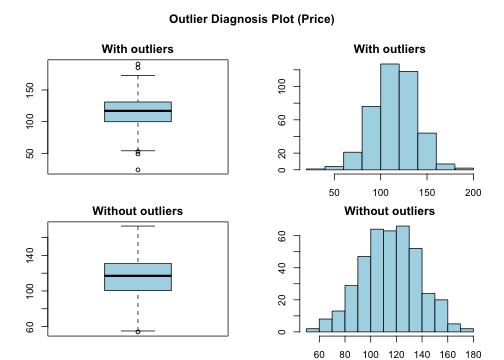### Reporting the information of data diagnosis for table of thr DBMS

The following shows several examples of creating an data diagnosis report for a DBMS table.

Using the `collect_size` argument, you can perform data diagonosis with the corresponding number of sample data. If the number of data is very large, use `collect_size`.

``````# create pdf file. file name is DataDiagnosis_Report.pdf
con_sqlite %>%
tbl("TB_CARSEATS") %>%
diagnose_report()

# create pdf file. file name is Diagn.pdf, and collect size is 350
con_sqlite %>%
tbl("TB_CARSEATS") %>%
diagnose_report(collect_size = 350, output_file = "Diagn.pdf")

# create html file. file name is Diagnosis_Report.html
con_sqlite %>%
tbl("TB_CARSEATS") %>%
diagnose_report(output_format = "html")

# create html file. file name is Diagn.html
con_sqlite %>%
tbl("TB_CARSEATS") %>%
diagnose_report(output_format = "html", output_file = "Diagn.html")
``````